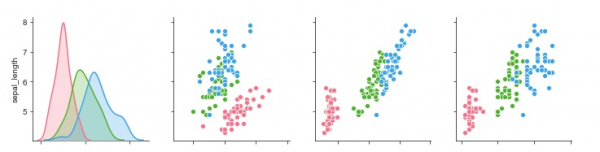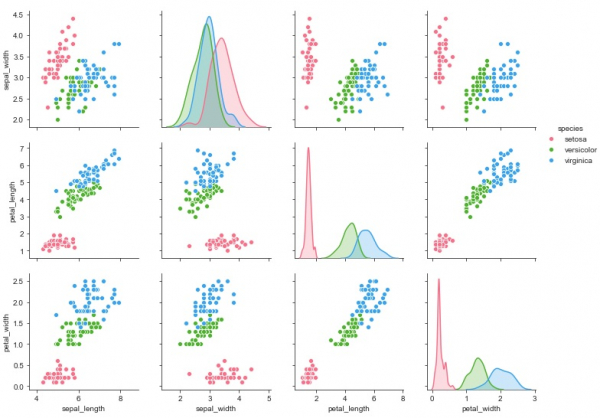# How can data that has multiple variables be visualized using Seaborn in Python?

Seaborn is a library that helps in visualizing data. It comes with customized themes and a high level interface. In real-time situations, dataset contains many variables. Sometimes, it may be required to analyse the relationship of every variable with every other variable in the dataset. In such situations, bivariate distribution may take up too much time and may get complicated as well.

This is where multiple pairwise bivariate distribution comes into picture. The ‘pairplot’ function can be used to get the relationship between combinations of variables in a dataframe. The output would be a univariate plot.

Syntax of pairplot function

seaborn.pairplot(data,…)

Now let us understand how it can be plotted on a graph −

## Example

import pandas as pd
import seaborn as sb
from matplotlib import pyplot as plt
sb.set_style("ticks")
sb.pairplot(my_df,hue = 'species',diag_kind = "kde",kind = "scatter",palette = "husl")
plt.show()

## Output## Explanation

• The required packages are imported.
• The input data is ‘iris_data’ which is loaded from the scikit learn library.
• This data is stored in a dataframe.